# Detection of recurrent rearrangement breakpoints from copy number data

## Abstract

### Background

Copy number variants (CNVs), including deletions, amplifications, and other rearrangements, are common in human and cancer genomes. Copy number data from array comparative genome hybridization (aCGH) and next-generation DNA sequencing is widely used to measure copy number variants. Comparison of copy number data from multiple individuals reveals recurrent variants. Typically, the interior of a recurrent CNV is examined for genes or other loci associated with a phenotype. However, in some cases, such as gene truncations and fusion genes, the target of variant lies at the boundary of the variant.

### Results

We introduce Neighborhood Breakpoint Conservation (NBC), an algorithm for identifying rearrangement breakpoints that are highly conserved at the same locus in multiple individuals. NBC detects recurrent breakpoints at varying levels of resolution, including breakpoints whose location is exactly conserved and breakpoints whose location varies within a gene. NBC also identifies pairs of recurrent breakpoints such as those that result from fusion genes. We apply NBC to aCGH data from 36 primary prostate tumors and identify 12 novel rearrangements, one of which is the well-known TMPRSS2-ERG fusion gene. We also apply NBC to 227 glioblastoma tumors and predict 93 novel rearrangements which we further classify as gene truncations, germline structural variants, and fusion genes. A number of these variants involve the protein phosphatase PTPN12 suggesting that deregulation of PTPN12, via a variety of rearrangements, is common in glioblastoma.

### Conclusions

We demonstrate that NBC is useful for detection of recurrent breakpoints resulting from copy number variants or other structural variants, and in particular identifies recurrent breakpoints that result in gene truncations or fusion genes. Software is available at http://http.//cs.brown.edu/people/braphael/software.html.

## Background

Copy number variants (CNVs) are genomic rearrangements that result in a different number of copies of a segment of the genome, and include deletions, amplifications, and unbalanced translocations. CNVs are common in the human genome, and CNVs have been associated with several diseases . Similarly, CNVs (also referred to as copy number aberrations, or CNAs) are found in many cancer genomes [4, 5]. Thus, detection of CNVs and characterization of the gene or genes that they affect is an important task.

Array comparative genome hybridization (aCGH)  is a widely-used experimental technique for the measurement of copy number variants in genomes. aCGH involves the hybridization of differentially fluorescently labeled DNA fragments from a test genome and a reference genome to a set of genomic probes derived from the reference genome sequence. Measurements of the test:reference fluorescence ratio at each probe identify locations in the test genome that are present in lower, higher, or similar copy in the reference genome, producing a copy number profile of the test genome. Copy number profiles are typically compared across individuals to identify recurrent CNVs that are shared by multiple individuals. These recurrent CNVs may be germline polymorphisms, or in the case of cancer samples, recurrent somatic mutations. Large cohorts of aCGH data from cancer genomes (e.g. from The Cancer Genome Atlas (TCGA) ) provide the statistical power to identify numerous recurrent somatic CNVs. Several methods have been introduced to identify recurrent CNVs, including GISTIC , CoCoA , STAC , and CMDS . These methods (with the exception of CMDS) first partition each copy number profile into regions (or segments) of equal copy number, producing a segmentation for each individual (see  for a survey of segmentation methods). Since a CNV alters the copy number of multiple adjacent probes, segmenting the copy number profile helps overcome experimental errors at each probe. These segmentations are then combined to identify aberrant intervals that are shared by multiple individuals. An implicit assumption of this approach is that the target of the CNV lies within the interval; this is the case for oncogenes that lie within amplifications or tumor suppressor genes that lie within deletions.

Some recurrent rearrangements do not target a gene within the aberrant interval, but rather target a gene or locus at the boundary of the interval. A striking example is the TMPRSS2-ERG fusion gene in prostate cancer . This fusion gene results from a 3 Mb deletion on chromosome 21, where the two endpoints (or breakpoints) of the deletion lie in the two partner genes of the fusion. More recently, next-generation DNA sequencing has shown other fusion genes that are located at the endpoints of the CNVs (cf. figure two (b) in ). These and other examples motivate the development of methods that discover recurrent breakpoints rather than recurrent intervals.

We introduce a novel algorithm called Neighborhood Breakpoint Conservation (NBC) to identify recurrent breakpoints in copy number data. NBC computes the probability that a breakpoint occurs between each pair of adjacent probes over all possible segmentations of a single copy number profile and then combines these probabilities across multiple profiles to identify recurrent breakpoints. The probabilistic approach contrasts with the typical methods for aCGH analysis that compute only a single segmentation of a copy number profile. Consideration of a single segmentation is reasonable for identifying recurrent aberrations because large aberrations will typically overlap in different individuals as long as the segmentations reasonably approximate the true underlying copy number level. However, identification of recurrent breakpoints is more sensitive to the choice of segmentation. Due to measurement errors in individual probes, the optimal segmentation of each individual profile may not "align" across profiles. Thus it is necessary to consider multiple suboptimal segmentations. Moreover the probabilistic approach allows use to account for biological variability in the location of a breakpoint within a gene or other locus. We apply NBC to aCGH data from 36 primary prostate tumors and predict 12 CNVs, including one gene truncation and one fusion gene which is the well-known TMPRSS2-ERG fusion gene. We also apply NBC to 227 glioblastoma (GBM) tumors and predict 91 CNVs, including 23 gene truncations and 33 fusion genes. Additionally, we predict 35 germline CNVs from 107 available matched blood samples from GBM patients. A number of the somatic CNV predictions in GBM involve the protein phosphatase PTPN12, suggesting that deregulation of PTPN12 via a variety of rearrangements is common in glioblastoma. We note that NBC is readily adapted to analyze copy number profiles obtained from next-generation DNA sequencing data [17, 18].

## Methods

The Neighborhood Breakpoint Conservation (NBC) algorithm takes, as input, aCGH data from many individuals and identifies recurrent breakpoints and pairs of recurrent breakpoints in a subset of the individuals (Figure 1). The first step in NBC, as in most aCGH analysis, is to segment each copy number profile into intervals of equal copy number.

While many existing methods produce a single segmentation for aCGH data , NBC uses a dynamic programming approach  to compute the probability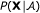of a copy number profile X given a segmentation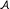. NBC then employs a stochastic backtrace to compute the posterior probability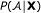. Using this approach, one can derive the segmentation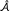with maximum probability, but more importantly, one can compute the posterior probability of events of interest over all possible segmentations of the data. In particular, we compute the probability of a breakpoint between each pair of adjacent probes, as well as the probability of a breakpoint within a fixed interval or probes (e.g. from a gene region).

The second step of NBC is to combine breakpoint probabilities in each individual to determine breakpoints that appear in multiple individuals. Similar to , we use a binomial order statistic  to compute a p-value for the event that k or more individuals share a breakpoint between two adjacent probes. We then extend this breakpoint score to consider pairs of breakpoints that are shared by multiple individuals. Finally, we also define a score for a breakpoint that may occur anywhere within an interval of adjacent probes (e.g. a gene) that is shared by multiple individuals. We detail each of these two steps in the following sections.

### A Probability Model for Segmentation and Breakpoint Analysis

A probabilistic formulation of the segmentation problem assigns a probability to each possible segmentation of X. The probability of other events, such as a breakpoint occurring at a particular locus, are readily computed from this model. Probabilistic segmentation approaches have been previously applied to CNV detection [21, 2426], but we found that these methods either: require a finite number of copy number levels (as in the Bayesian Hidden Markov method of ); focus on probabilistic model selection rather than an explicit probabilistic model for the segmentation itself ; or do not perform well on high-resolution oligonucleotide arrays (see Additional File 1, Figure S3 for a comparison to ).

Our algorithm is based on the change-point model described in . Consider a copy number profile X = (X1,...,X n ), where X i is the log2 ratio of test.reference DNA at the i th probe. We assume that the test genome consists of an unknown number of segments K with corresponding copy numbers Θ = {θ1,...,θ K }. Following the usual assumptions for aCGH data [20, 21, 2426], we assume that each X i is normally distributed with mean μ i and variance σ2. The variance σ2 is a hyperparameter whose value must be set. Below we describe how we estimate this value from the data. The mean μ i equals θ s if probe i lies within segment s. Further, we assume that X i from different segments are independent. Let l j denote the number of probes in segment j, and let kmax denote the maximum number of segments in the test genome.

We define the breakpoint sequence A = (A1, ..., AK+1), where A v is the index of the probe at the start of the v + 1st segment and AK+1= n is a "dummy" breakpoint signifying the end of the sequence (i.e. there are K+1 breakpoints representing K segments in A). Thus,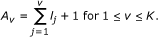The unknowns in our model are the breakpoint sequence A, the number of segments K, and the segment copy numbers Θ. We assume a priori that Θ is independent of A and K. We further assume that the segment copy numbers θ s Θ are independent and normally distributed with mean μ0 and variance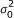. (The assumption that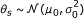gives a conjugate prior for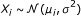allowing us to compute some probabilities analytically. See Additional File 1, Section SA.) We assign a prior on breakpoints sequences A such that all A with K segments are equally likely,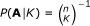. Additionally, we assign a prior on the number of segments K such that there is a probability ofof a single segment (K = 1) and the remaining values of K, 1 < Kkmax, are equally likely. Note that these priors do not make any strong assumptions about the data. essentially, the a priori assumption is that with probabilitythe data is produced from a single segment.

From the priors P (A|K) and P (K = k) and the values of the hyperparameter σ; μ0, σ0, the joint distribution P (X, A, Θ, K) can be derived (Additional File 1, Section SA).

#### Hyperparameter Estimation

The segmentation and breakpoint analysis algorithm relies on setting values for the hyperparameters μ0 (the baseline mean),(the variance in segment copy numbers), and σ2 (the variance in probe measurements). We describe how to estimate these from the copy number profile X = (X1,...,X n ). First, we set μ0 to be the median of the X i . To estimate the variancesand σ2, we form sliding windows of 10 probes. Let V be the median of the sample variances of the windows, and let M be the maximum absolute difference between the sample means of the windows and μ0. We set the measurement variance σ2 = 2V and the segment variance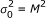.

To test the sensitivity of our results to our particular estimates of the hyperparameters - in particular our estimates of σ2 and- we performed two simulations that are inspired by the simulations of .

Simulation #1 We generated an artificial chromosome with 100 probes containing a 40 probe single-copy gain (log2 ratio of 1) placed in the center. We then introduced various amounts of gaussian noise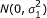in the probe measurements, setting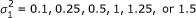. For each value of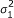, we generated 100 such chromosomes.

Simulation #2 We generated an artificial chromosome with 100 probes with gaussian noise N(0, 0.5) in the probe measurements. We then introduced a 40 probe aberration at various log2 ratios. 0.5, 1, 2, 3, 4, 5, and 6. For each log2 ratio, we generated 100 such chromosomes.

A representative sample of the datasets for Simulation #1 and Simulation #2 are shown in Additional File 1, Figure S1 and S2.

We ran NBC on datasets from the two simulations with different estimates for the variancesand σ2, detailed below. To assess the quality of the resulting breakpoint predictions, we consider probe locations with Pr(breakpoint) ≥ 0.5 to be a predicted breakpoint. We assume that a predicted breakpoint detects a true breakpoint if the predicted breakpoint location is ≤ 2 probes away from the true breakpoint location. We count the number of true positive predictions (0, 1, or 2). Additionally, we count the number of false positive predictions for each dataset. We average the true positives and false positives over the 100 artificial chromosomes.

Simulation #1 has a fixed aberration log2 ratio, so we set the segment varianceand we test three different values of σ2: V, 2V, and 3V (Figure 2 top row). Compared to our estimated value of σ2 = 2V, the number of true positives is similar when σ2 = V or σ2 = 3V and the measurement erroris low. Asincreases, setting σ2 = V results in more false positives compared to our estimate σ2 = 2V, while setting σ2 = 3V results in fewer total predictions, including true positives. Thus, at lower measurement error the results are not particularly sensitive to the value of σ2, with our estimate σ2 = 2V maintaining reasonable sensitivity and specificity and higher measurement error.

Since Simulation #2 has fixed measurement error, we set the measurement variance σ2 = 2V and test three different values of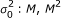, and M3 (Figure 2 bottom row). The number of true and false positives is very similar for all three estimates of. The only exception is that when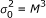, there is a large variation in the number of false positives over the different simulated chromosomes. These simulations show that our hyperparameter estimates are reasonable, although other estimation approaches are possible.

The simulations underscore that the ability to detect the breakpoints of a segment is related to both the copy number of the segment (governed by the segment variance) and the measurement error (governed by the variance σ2). For example, in Simulation #1 (whereis fixed), as the probe variance σ2 increases the average number of false positive breakpoints increases while the average number of true positives remains below one. To avoid such situations, we do not segment the data and immediately report 0 breakpoints when our estimates of σ and σ0 satisfy σ ≥ 3σ0.

#### Computing Breakpoint Probabilities

We compute the probability of a breakpoint between pairs of adjacent probes by sampling breakpoint sequences A from the distribution P (A|X) and counting the proportion of samples that have a breakpoint between adjacent probes. Note that the probability of a breakpoint between adjacent probes can be analytically computed (see ). We describe a sampling strategy, since this will generalize to the computation of the probability of breakpoints that lie within an interval or pairs or breakpoints. For notational convenience, let

X[i:j]= (X i ,..., X j ), X(i:j](Xi+1,..., X j ), and X[i:j)= (X i ,..., Xj-1) The probability of X is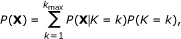(1)

where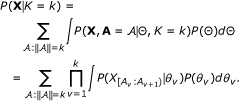(2)

Here,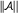is the length (number of breakpoints) ofP (X|K = k) is the probability of the data X given that the test genome is divided into k segments, and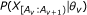is the probability that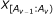consists of a single segment. The product in Equation (2) results from the segment independence assumption. The choice of a conjugate prior for P (θ) allows the integral to be analytically computed (Additional File 1, Section SA.2). However, calculating P (X|K = k) in this way requires summing over all possible breakpoint sequences A and is computationally infeasible. A dynamic program allows the efficient computation of this term.

#### Dynamic program

Let P (X[i:j]|k) be the probability of observing X[i:j]given that it is generated from k different segments. We compute this P (X[1:j]|k) for 1 ≤ kkmax and 1 ≤ jn as follows.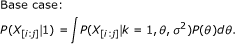(3)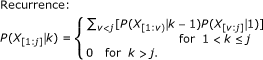(4)

The final row of the dynamic programming table contains P (X|K = k) for 1 ≤ kkmax, which is used in Equation (1) to compute P (X).

#### Recursive sampling

We use P (X|K = k) as well as the base case P (X[i:j]|1) and intermediate terms P (X[1:j]|k) in the dynamic program to sample exact and independent breakpoint sequences A using a backward sampling technique .

1. 1.

Draw K = k from P (K = k|X), determined by inverting P (X|K = k) using Bayes Rule.

2. 2.

Set A k+1= n.

3. 3.

Draw A k , A k-1, ..., A 1 recursively using the conditional distributions computed by the recurrences in Equation (4). Given A q , the location of the beginning of the q th segment, the distribution of A q-1is obtained as follows.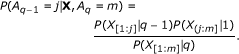(5)

From a set of breakpoint sequences sampled in proportion to P (A|X), we determine the probability of a breakpoint occurring between two adjacent probes by counting the proportion of samples that contain a breakpoint at that locus. Other probabilities derived from these sampled breakpoint sequences are described in subsequent sections.

#### Runtime analysis

The base cases P (X[i:j]|1) require O(n2) computations and the dynamic program requires O(nkmax) computations; thus computing P (X|K = k) is achieved in O(n(n + kmax)) time. All computations necessary to sample a breakpoint sequence A are already computed in the dynamic program, so sampling is linear in the number of breakpoints K drawn from P (X|K = k).

### Identifying Recurrent Breakpoints

After sampling breakpoint sequences for a set of individuals, we identify recurrent breakpoints that appear in many individuals at the same genomic locus. Let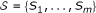be a set of copy number profiles from m individuals, where S j = (X1, ..., X n ) is the copy number profile for individual j. We assume that the same array probes are used for each individual, i.e. the i th probe in individual S j is at the same location as the i th probe in individual S j' . We analyze recurrent breakpoints at two levels of resolution.

• Recurrent probe breakpoints occur between the same two array probes in a subset of individuals.

• Recurrent interval breakpoints occur within the same interval of the genome in a subset of individuals.

In addition to analyzing these types of recurrent breakpoints, we also consider pairs of recurrent breakpoints to identify recurrent CNVs. Note that these pairs may indicate intrachromosomal CNVs, as in the case of classic copy number aberrations like duplications and deletions, or interchromosomal CNVs, as in the case of (unbalanced) translocations.

#### Recurrent probe breakpoints

For each probe, we define a score that measures the presence of a breakpoint in a subset of individuals. We design this score to account for the observation that the number of breakpoints in copy-number profiles, particularly in a set of cancer samples, is highly variable. That is, in a set of cancer samples, even from the same cancer type, there will typically be highly rearranged cancer genomes with many breakpoints, and less rearranged genomes with relatively few breakpoints. This variability in the number of breakpoints is maintained following our Bayesian segmentation approach - despite the fact that we use the same flat prior for each individual - because there is strong evidence to support a larger number of breakpoints in some samples. Since there is a greater chance of recurrent breakpoints occurring randomly in a collection of highly rearranged genomes than a collection of less rearranged genomes, it is advantageous to consider the number of breakpoints in each profile when scoring recurrent breakpoints. Because the variability of number of breakpoints across different individuals is typically not well matched by a standard distribution, one approach is to use a permutation test that preserves the number and probability of breakpoints in each profile while permuting their location. We instead derive a score for recurrent probe breakpoints based on a binomial order statistic [11, 23]. This score first normalizes the breakpoint probability at each probe in each individual according to the breakpoint probabilities across all probes in individual. These normalized values are then combined across multiple individuals to produce a recurrent breakpoint score.

Let b i be the event that a breakpoint lies between probes i and i + 1; P (b i |S j ) is the breakpoint probability at probe i in individual S j , and is computed by counting the proportion of sampled breakpoint sequences A that have a breakpoint between i and i + 1. Let ρ j (i) be the fraction of probes with a higher breakpoint probability than probe i in individual S j (the normalized rank of probe i).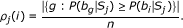(6)

Let π be a permutation of the individuals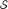such that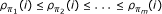. For 1 ≤ hm, we wish to determine the probability that h or more individuals have a breakpoint at location i. Because of our normalization of the breakpoint probabilities in each sample, under the null hypothesis the individual scores ρ j (i) are independent and uniformly distributed in [0,1]. Thus, the probability that h or more individuals have a breakpoint at location i is given by the tail of the binomial distribution with success probability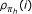. The p-value for the probe location i is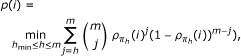(7)

where we are only interested in scoring those breakpoints that are present in at least hmin patients. Note that because the binomial order statistic is computed from the empirical distribution ρ j of breakpoint probabilities in each sample, the relative magnitude of the breakpoint probability is not used in the computation. Despite this loss of information, we found that the binomial order statistic produced reasonable results on real data (See Results below) and was more efficient than a permutation test.

Finally, we assume that a recurrent breakpoint is also conserved in the direction of the copy number change: all samples with a recurrent breakpoint are either breakpoints that go from relatively low copy number to high copy number of vice versa. A breakpoint sequence A defined a segmentation, and we use the mean values of each segment to determine the direction of copy number change. The copy number change is positive if the mean of the segment to the right of the breakpoint is higher than the mean of the segment to the left. We test both cases for each recurrent breakpoint, doubling the number of hypotheses we test. We control the False Discovery Rate (FDR) using the method of Benjamini and Hochberg .

#### Recurrent interval/gene breakpoints

We extend our approach to find recurrent breakpoints that lie within a genomic interval W; e.g. a gene. Unlike the recurrent probe breakpoint calculation above, where each probe was a priori equally likely to contain a breakpoint, intervals that contain more probes are a priori more likely to contain a breakpoint than intervals that contain fewer probes. To account for this, we use a log-odds score that is defined as follows. Let b W be the event that one or more breakpoints lie between any pair of adjacent probes within W. Similarly, let b W be the event that no breakpoint lies between any adjacent probes within W. The log-odds score ℓ j (W) that patient S j contains a breakpoint within W is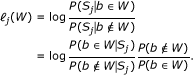(8)

The conditional probabilities P(b W |S j ) and P(b = W |S j ) describe probabilities over all possible segmentations of the copy number profile S j . P(b W |S j ) is determined by sampling breakpoint sequences A and counting the number of samples that contain one or more breakpoints in the interval W. P(b = W |S j ) is then simply 1- P(b W |S j ). The scaling factor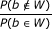is computed by counting the number of ways to place breakpoints such that none of them lie in W: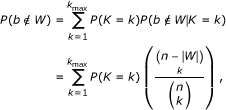(9)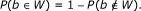(10)

Here, the last term in Equation (9) counts the number of ways to choose k breakpoints that do not lie in W. As in the recurrent breakpoint computation above, we use the binomial order statistic to combine log-odds scores across patients. First, in an analogous computation to Equation (6) we normalize the log-odds scores using the empirical cumulative distribution, which produces the normalized rank of ℓ j (W) for all j: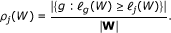(11)

Finally, using the ρ j (W ) scores for each patient S j we compute the p-value ρ(W ) using the binomial order statistic as in Equation (7).

For the experiments below, we define the the copy number change for an interval W to be positive if at least 90% of the breakpoints within the interval are positive and negative if at least 90% of the breakpoints within the interval are negative. Otherwise, we do not call a breakpoint in W.

#### Pairs of recurrent interval/gene breakpoints

We identify pairs of non-overlapping recurrent interval breakpoints using a log-odds score similar to Equation (8) that scores two breakpoints occurring in intervals W1 and W2. An important case we will consider is when W1 and W2 are genes. Let b W1 be the event that a breakpoint lies between any pair of adjacent probes within W1, and Let b' W2 be the event that a breakpoint lies between any pair of adjacent probes within W2. We define the score for intervals W1 and W2 for a particular patient S j .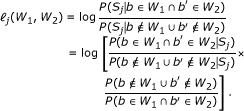(12)

Each term is computed similarly to Equation (8). If W1 and W2 are on different chromosomes, the events P (b W1) and P (b' W2) are independent and Equations (9) and (10) are used to compute the scaling factor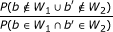. If the intervals are on the same chromosome then the events are dependent, and the numerator in the scaling factor is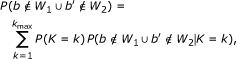(13)

Where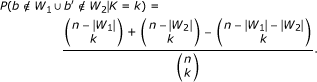The denominator in the scaling factor is then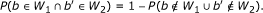The p-value ρ(W1, W2) is computed by normalizing as in Equation (11) according to the empirical distribution of log-odds scores over all pairs of non-overlapping intervals and then using the binomial order statistic to determine the final p-value. Here, we test four hypotheses for each pair W1 and W2 by considering the four combinations of direction of copy number change: {(+, +), (-, -), (-, +), (-, -)}. Note that restricting W1 and W2 to each contain a single probe identifies pairs of recurrent probe breakpoints.

### Predicting Structural Variants, Gene Truncations, and Fusion Genes

Our statistics for single recurrent breakpoints (ρ(i) and ρ(W)) and pairs of recurrent breakpoints (ρ(i, j) and ρ(W1, W2)) provide a flexible framework to predict particular rearrangement configurations. In this paper, we classify predictions into structural variants, gene truncations, and fusion genes.

#### Structural variants

Pairs of recurrent probe breakpoints may indicate germline or somatic rearrangements that have recurrent breakpoints at the highest resolution allowed by the spacing of probes. To identify these rearrangements, we compute the pairs of recurrent probe breakpoint statistic for every pair of probes within each chromosomal arm. Note that this limits the structural variant predictions to intrachromosomal rearrangements only.

#### Gene truncations

Recurrent breakpoints found within a single gene may indicate a gene truncation, resulting in the loss of functionality for a particular gene. To predict gene truncations, we compute the recurrent interval breakpoint detection statistic, using the set of gene regions from RefSeq as our intervals of interest.

#### Fusion genes

Pairs of recurrent interval breakpoints found within genes suggest potential fusion genes. We compute pairs of recurrent interval breakpoints using all pairs of gene regions from RefSeq as our intervals of interest. Note that not all pairs of recurrent genes suggest functional fusion genes. For example, a rearrangement that joins the 3' end of one gene to the 3' end of another gene is typically not a functional fusion gene. Thus, we restrict our attention to pairs of interval breakpoints with particular configurations (Figure 3).

Specifically, consider a pair of recurrent intervals G1 and G2 that represent gene regions. Each gene has an orientation, orient(G1) {+, -} and orient(G2) {+, -}. Additionally, the breakpoint that lies within each recurrent interval has an associated direction of copy number change, dir(G1) {+, -} and dir(G2) {+, -}. We assume that a fusion gene contains the 5' end of one gene joined to the 3' end of the other gene and thus satisfies the following rule.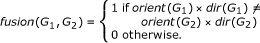(14)

### Filtering and Ranking Predictions

We apply a number of additional steps to remove and prioritize predictions. In the case of fusion genes, if there are many predictions remaining we rank these predictions by the preservation of copy number across the fusion point.

#### Removing single probe aberrations

Single probe aberrations are segments consisting of a single probe. Since these are difficult to distinguish from experimental artifacts, we remove them from further consideration. Single probe aberrations are characterized by two large changes in copy number in adjacent probes, where the segments adjacent to this aberration have a similar copy number. We identify these probes and remove them from the analysis.

#### Removing known CNVs

We remove predictions that are new known CNVs. We say that a single probe is "near" a known CNV in the Database of Genomic Variants (DGV)  if it is within 10 kb of a recorded copy number variant endpoint, and a gene region is "near" a known copy number variant if it is within 10 kb of a recorded copy number variant endpoint. Additionally, a pair of intrachromosomal recurrent breakpoints are near a variant if at least one of the breakpoints is within 10 kb of a recorded copy number variant endpoint and the mutual overlap between the prediction interval (defined by the pair of breakpoints) and the variant interval is greater than 50%.

#### Ranking predictions

Since fusion genes (and other recurrent pairs of breakpoints) are physically joined in the test genome, we expect the copy number of either side of the breakpoint to be the same. Thus, we rank these predictions by calculating the root mean squared difference (RMS) between the copy number levels of probes surrounding the breakpoint. Consider fusion gene predictions. we know the configuration of the gene partners, but we do not know exactly where the breakpoint lies. Thus, we determine the copy number on each side of the fusion as the average of the three flanking probes of the left gene partner and the three flanking probes of the right gene partner. If h patients have the breakpoint, determined by the argmax of Equation (7),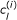is the left-flanking copy number of the fusion and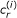is the right-flanking copy number of the fusion, then the RMS difference of the pair of conserved breakpoints is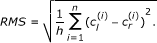(15)

## Results

We applied NBC to two aCGH datasets. a collection of 36 primary prostate tumors, and 227 glioblastoma (GBM) tumors. For each dataset, we computed recurrent probe breakpoints, recurrent gene breakpoints, pairs of recurrent probe breakpoints, and pairs of recurrent gene breakpoints.

### Prostate Dataset

We applied NBC to Agilent aCGH data from a collection of 36 primary prostate tumors. Each sample contained copy number ratios for 235,719 aCGH probes that were mapped to the hg17 human reference genome. We examined recurrent gene breakpoints using the gene regions from 16,162 hg17 RefSeq genes. Table 1 reports the number of predicted variants, and tables listing the breakpoint coordinates and additional information are in Additional File 2, Tables S1, S2, S3 and S4. We visualize predictions by plotting the average segmentation for each of the individuals that were involved of the final p-value computations for recurrent breakpoints in Equation (7). The average segmentation is created by averaging the segment copy numbers Θ at each probe for the sampled breakpoint sequences A. We predict one novel gene truncation, which occurs in the Complement factor H (CFH) gene (Figure 4). CFH encodes a protein that is secreted into the bloodstream and is essential for complement system regulation, and CFH polymorphisms are associated with macular degeneration . From pairs of recurrent probes, we predict 10 novel variants, the most significant of which lies in the DEFB locus ((p-value = 1.3 × 10-33, Figure 5). DEFB genes have been associated with the risk of prostate cancer , and this locus lies near many known CNVs, complicating the study of nearby genes. We predict only one fusion gene, the well-known TMPRSS2-ERG fusion gene, which we detect in 5 patients with a p-value of 2.7 × 10-10 (Figure 6). The TMPRSS2-ERG fusion gene has an RMS difference of 0.2520.

#### Comparison to Segmentation Approaches

To demonstrate the importance of breakpoint uncertainty in computing recurrent breakpoints, we compared our fusion gene predictions to those obtained using a single segmentation for each individual. We segmented copy number profiles from each individual using Circular Binary Segmentation (CBS)  (Additional File 1, Section SB). CBS returns a single segmentation (and thus a set of breakpoints) for each individual. From these sets of breakpoints, for each pair of genes from the same chromosome, we counted the number of patients with a breakpoint in each gene. Only two individuals had a pair of breakpoints within TMPRSS2 and ERG from the CBS segmentations (Additional File 1 Figure S4). Further, there are 5 fusion gene predictions that occur in two individuals after applying the filters described previously, and zero predictions that occur in more than two individuals. Since no other common fusion genes in prostate cancer are known, we assume that these remaining predictions are false positives. Thus, NBC is more sensitive and specific in fusion gene identification.

### Glioblastoma Dataset

We next applied our method to Agilent 244 K aCGH data of glioblastoma (GBM) tumors from The Cancer Genome Atlas . Data was collected from 233 GBM patients, including 227 tumor samples and 107 matched blood samples. Each sample contains 227,612 aCGH probes across the hg18 human reference genome. Gene regions from 16,162 hg18 RefSeq genes were used to determine recurrent gene breakpoints. Classification of breakpoints in the tumor samples and filtering of the predictions were performed as above. Additionally, to restrict attention to somatic breakpoints we remove from consideration any recurrent breakpoints found in the tumor samples that also appear in the blood samples. When identifying recurrent probe breakpoints in the blood samples, we increase the False Discovery Rate (FDR) from 0.01 to 0.1 to more aggressively filter recurrent breakpoints in tumor samples. Table 2 reports the number of predicted variants, and tables listing the breakpoint coordinates and additional information are in Additional File 2, Tables S5, S6, S6 and S8.

We predict 23 gene truncations from the tumor samples, three of which are shown in Figure 7. Each of these has some support in the literature for an association with glioblastoma or other neuronal diseases. ECOP is co-amplified with EGFR in glioblastoma as well as other cancers [31, 32], RUNX2 is expressed in glioblastoma cells , and PCDH11X is associated with late-onset Alzheimer's disease . We also predict 33 fusion genes from the tumor samples. One of these predictions involving INTS2 and MED13 might arise due to a tandem duplication whose breakpoints are within the two genes (Figure 8a). Another prediction involves PPP1R9A, which is an imprinted gene that appears in neuronal tissues and has been shown to be expressed in other embryonic tissues  (Figure 8b). The phosphatase PTPN12 appears highly rearranged in 16 GBM patients, and it is a partner in a surprisingly large fraction (11/33) of the fusion gene predictions (Table 3). PTPN12 is known to dephosphorylate oncogenes c-ABL and Src; thus deregulation of PTPN12 might contribute to tumor survival . While the 5' end of PTPN12 appears amplified with respect to the log2 copy number ratios at the 3' end, many fusion gene predictions consist of a deletion of the 3' end (i.e. Figure 9a). Additionally, some fusion gene candidates might indicate multiple rearrangements, such as a translocation occurring after an amplification that results in a fusion gene configuration (Figure 9b). Due to the large number of candidate rearrangement partners of PTPN12, it might be the deregulation of PTPN12, and not necessarily any single rearrangement, that is important for GBM.

## Discussion

NBC successfully identifies known fusion genes and structural variants. For fusion genes, NBC's consideration of uncertainty and variability in the locations of breakpoints provides an advantage over methods that compare individual segmentations of copy number profiles. This advantage is mitigated for variants with highly conserved breakpoints such as germline structural variants that are common in a population. However, it is possible that NBC would be helpful for complex, or overlapping, structural variants, where recurrent breakpoints might be a stronger signal than recurrent aberrant intervals.

NBC relies on a Bayesian change point algorithm, which requires specifying both prior distributions and a few hyperparameters. The weak priors that we use do not make strong assumptions about the data. However, hyperparameter estimation for Bayesian change point algorithms remains a difficult problem, and is sensitive to the particular type of data to be segmented. While our method chooses the hyperparameters systematically from the data rather than requiring user-defined input, poor parameter estimation leads to excessive breakpoint calling if there are no breakpoints to find or if the experimental error cannot be modeled by a constant σ2. We presented one approach to estimate hyperparameters from aCGH data, but more sophisticated methods (e.g. empirical Bayesian approaches) could be used .

In this paper, we focused on applications of NBC to aCGH data. But NBC is equally applicable to copy number profiles generated by mapping DNA sequence reads to a reference genome [17, 18]. With next generation sequencing technologies, breakpoint resolution can be much higher than most current aCGH methods, but the problems of breakpoint variability and uncertainty remain.

## Conclusions

We have introduced Neighborhood Breakpoint Conservation (NBC), an algorithm that identifies recurrent breakpoints in data from multiple individuals. NBC correctly identifies a known fusion gene (TMPRSS2-ERG) in aCGH data from 36 prostate tumors and predicts gene truncations, structural variants, and fusion genes in aCGH data from glioblastoma. We expect that application of our method to additional samples will allow us to uncover and categorize other recurrent germline and somatic rearrangements.

## References

1. Pinto D, et al.: Functional impact of global rare copy number variation in autism spectrum disorders. Nature 2010, 466: 368–372. 10.1038/nature09146

2. St Clair D: Copy number variation and schizophrenia. Schizophr Bull 2009, 35: 9–12. 10.1093/schbul/sbn147

3. Choy KW, Setlur SR, Lee C, Lau TK: The impact of human copy number variation on a new era of genetic testing. BJOG 2010, 117: 391–398. 10.1111/j.1471-0528.2009.02470.x

4. Pinkel D, Albertson DG: Array comparative genomic hybridization and its applications in cancer. Nat Genet 2005, 37(Suppl):S11–7.

5. Paris PL, Andaya A, Fridlyand J, Jain AN, Weinberg V, Kowbel D, Brebner JH, Simko J, Watson JE, Volik S, Albertson DG, Pinkel D, Alers JC, van der Kwast TH, Vissers KJ, Schroder FH, Wildhagen MF, Febbo PG, Chinnaiyan AM, Pienta KJ, Carroll PR, Rubin MA, Collins C, van Dekken H: Whole genome scanning identifies genotypes associated with recurrence and metastasis in prostate tumors. Hum Mol Genet 2004, 13: 1303–1313. 10.1093/hmg/ddh155

6. Pinkel D, Segraves R, Sudar D, Clark S, Poole I, Kowbel D, Collins C, Kuo WL, Chen C, Zhai Y, Dairkee SH, Ljung BM, Gray JW, Albertson DG: High resolution analysis of DNA copy number variation using comparative genomic hybridization to microarrays. Nat Genet 1998, 20(2):207–11. 10.1038/2524

7. Lucito R, Healy J, Alexander J, Reiner A, Esposito D, Chi M, Rodgers L, Brady A, Sebat J, Troge J, West JA, Rostan S, Nguyen KC, Powers S, Ye KQ, Olshen A, Venkatraman E, Norton L, Wigler M: Representational oligonucleotide microarray analysis: a high-resolution method to detect genome copy number variation. Genome Res 2003, 13(10):2291–305. 10.1101/gr.1349003

8. Barrett MT, Scheffer A, Ben-Dor A, Sampas N, Lipson D, Kincaid R, Tsang P, Curry B, Baird K, Meltzer PS, Yakhini Z, Bruhn L, Laderman S: Comparative genomic hybridization using oligonucleotide microarrays and total genomic DNA. Proc Natl Acad Sci USA 2004, 101(51):17765–70. 10.1073/pnas.0407979101

9. McLendon R, et al.: Comprehensive genomic characterization defines human glioblastoma genes and core pathways. Nature 2008, 455: 1061–1068. 10.1038/nature07385

10. Beroukhim R, Getz G, Nghiemphu L, Barretina J, Hsueh T, Linhart D, Vivanco I, Lee JC, Huang JH, Alexander S, Du J, Kau T, Thomas RK, Shah K, Soto H, Perner S, Prensner J, Debiasi RM, Demichelis F, Hatton C, Rubin MA, Garraway LA, Nelson SF, Liau L, Mischel PS, Cloughesy TF, Meyerson M, Golub TA, Lander ES, Mellinghoff IK, Sellers WR: Assessing the significance of chromosomal aberrations in cancer: methodology and application to glioma. Proc Natl Acad Sci USA 2007, 104: 20007–20012. 10.1073/pnas.0710052104

11. Ben-Dor A, Lipson D, Tsalenko A, Reimers M, Baumbusch LO, Barrett MT, Weinstein JN, Børresen-Dale AL, Yakhini Z: Framework for Identifying Common Aberrations in DNA Copy Number Data. RECOMB 2007 2007, LNBI(4453):122–136.

12. Diskin SJ, Eck T, Greshock J, Mosse YP, Naylor T, Stoeckert CJ, Weber BL, Maris JM, Grant GR: STAC: A method for testing the significance of DNA copy number aberrations across multiple array-CGH experiments. Genome Res 2006, 16: 1149–1158. 10.1101/gr.5076506

13. Zhang Q, Ding L, Larson DE, Koboldt DC, McLellan MD, Chen K, Shi X, Kraja A, Mardis ER, Wilson RK, Borecki IB, Province MA: CMDS: a population-based method for identifying recurrent DNA copy number aberrations in cancer from high-resolution data. Bioinformatics 2010, 26: 464–469. 10.1093/bioinformatics/btp708

14. Lai WR, Johnson MD, Kucherlapati R, Park PJ: Comparative analysis of algorithms for identifying amplifications and deletions in array CGH data. Bioinformatics 2005, 21(19):3763–70. 10.1093/bioinformatics/bti611

15. Tomlins SA, Rhodes DR, Perner S, Dhanasekaran SM, Mehra R, Sun XW, Varambally S, Cao X, Tchinda J, Kuefer R, Lee C, Montie JE, Shah RB, Pienta KJ, Rubin MA, Chinnaiyan AM: Recurrent fusion of TMPRSS2 and ETS transcription factor genes in prostate cancer. Science 2005, 310(5748):644–8. 10.1126/science.1117679

16. Campbell PJ, Stephens PJ, Pleasance ED, O'Meara S, Li H, Santarius T, Stebbings LA, Leroy C, Edkins S, Hardy C, Teague JW, Menzies A, Goodhead I, Turner DJ, Clee CM, Quail MA, Cox A, Brown C, Durbin R, Hurles ME, Edwards PA, Bignell GR, Stratton MR, Futreal PA: Identification of somatically acquired rearrangements in cancer using genome-wide massively parallel paired-end sequencing. Nat Genet 2008, 40: 722–729. 10.1038/ng.128

17. Chiang DY, Getz G, Jaffe DB, O'Kelly MJ, Zhao X, Carter SL, Russ C, Nusbaum C, Meyerson M, Lander ES: High-resolution mapping of copy-number alterations with massively parallel sequencing. Nat Methods 2009, 6: 99–103. 10.1038/nmeth.1276

18. Yoon S, Xuan Z, Makarov V, Ye K, Sebat J: Sensitive and accurate detection of copy number variants using read depth of coverage. Genome Res 2009, 19: 1586–1592. 10.1101/gr.092981.109

19. Olshen AB, Venkatraman ES, Lucito R, Wigler M: Circular binary segmentation for the analysis of array-based DNA copy number data. Biostatistics 2004, 5(4):557–572. 10.1093/biostatistics/kxh008

20. Picard F, Robin S, Lavielle M, Vaisse C, Daudin JJ: A statistical approach for array CGH data analysis. BMC Bioinformatics 2005, 6: 27. 10.1186/1471-2105-6-27

21. Zhang NR, Siegmund DO: A modified Bayes information criterion with applications to the analysis of comparative genomic hybridization data. Biometrics 2007, 63: 22–32. 10.1111/j.1541-0420.2006.00662.x

22. Liu JS, Lawrence CE: Bayesian inference on biopolymer models. Bioinformatics 1999, 15: 38–52. 10.1093/bioinformatics/15.1.38

23. David H, Nagaraja H: Order Statistics. 3rd edition. Edited by: Hoboken NJ. John Wiley; 2003.

24. Barry D, Hartigan JA: A Bayesian Analysis for Change Point Problems. Journal of the American Statistical Association 1993, 88(421):309–319. 10.2307/2290726

25. Erdman C, Emerson JW: A fast Bayesian change point analysis for the segmentation of microarray data. Bioinformatics 2008, 24: 2143–2148. 10.1093/bioinformatics/btn404

26. Guha S, Li Y, Neuberg D: Bayesian Hidden Markov Modeling of Array CGH Data. 2008, 103: 485–497.

27. Benjamini Y, Hochberg Y: Controlling the False Discovery Rate: A Practical and Powerful Approach to Multiple Testing. Journal of the Royal Statistical Society. Series B (Methodological) 1995, 57: 289–300.

28. Iafrate AJ, Feuk L, Rivera MN, Listewnik ML, Donahoe PK, Qi Y, Scherer SW, Lee C: Detection of large-scale variation in the human genome. Nat Genet 2004, 36: 949–951. 10.1038/ng1416

29. Christen WG, Glynn RJ, Chew EY, Albert CM, Manson JE: Folic acid, pyridoxine, and cyanocobalamin combination treatment and age-related macular degeneration in women: the Women's Antioxidant and Folic Acid Cardiovascular Study. Arch Intern Med 2009, 169: 335–341. 10.1001/archinternmed.2008.574

30. Huse K, Taudien S, Groth M, Rosenstiel P, Szafranski K, Hiller M, Hampe J, Junker K, Schubert J, Schreiber S, Birkenmeier G, Krawczak M, Platzer M: Genetic variants of the copy number polymorphic beta-defensin locus are associated with sporadic prostate cancer. Tumour Biol 2008, 29: 83–92. 10.1159/000135688

31. Eley GD, Reiter JL, Pandita A, Park S, Jenkins RB, Maihle NJ, James CD: A chromosomal region 7p11.2 transcript map: its development and application to the study of EGFR amplicons in glioblastoma. Neurooncology 2002, 4: 86–94.

32. Baras A, Yu Y, Filtz M, Kim B, Moskaluk CA: Combined genomic and gene expression microarray profiling identifies ECOP as an upregulated gene in squamous cell carcinomas independent of DNA amplification. Oncogene 2009, 28: 2919–2924. 10.1038/onc.2009.150

33. Vladimirova V, Waha A, Luckerath K, Pesheva P, Probstmeier R: Runx2 is expressed in human glioma cells and mediates the expression of galectin-3. J Neurosci Res 2008, 86: 2450–2461. 10.1002/jnr.21686

34. Carrasquillo MM, Zou F, Pankratz VS, Wilcox SL, Ma L, Walker LP, Younkin SG, Younkin CS, Younkin LH, Bisceglio GD, Ertekin-Taner N, Crook JE, Dickson DW, Petersen RC, Graff-Radford NR, Younkin SG: Genetic variation in PCDH11X is associated with susceptibility to late-onset Alzheimer's disease. Nat Genet 2009, 41: 192–198. 10.1038/ng.305

35. Nakabayashi K, Makino S, Minagawa S, Smith AC, Bamforth JS, Stanier P, Preece M, Parker-Katiraee L, Paton T, Oshimura M, Mill P, Yoshikawa Y, Hui CC, Monk D, Moore GE, Scherer SW: Genomic imprinting of PPP1R9A encoding neurabin I in skeletal muscle and extra-embryonic tissues. J Med Genet 2004, 41: 601–608. 10.1136/jmg.2003.014142

36. Meng F, Henson R, Lang M, Wehbe H, Maheshwari S, Mendell JT, Jiang J, Schmittgen TD, Patel T: Involvement of human micro-RNA in growth and response to chemotherapy in human cholangiocarcinoma cell lines. Gastroenterology 2006, 130: 2113–2129. 10.1053/j.gastro.2006.02.057

37. Lian H, Thompson WA, Thurman R, Stamatoyannopoulos JA, Noble WS, Lawrence CE: Automated mapping of large-scale chromatin structure in ENCODE. Bioinformatics 2008, 24: 1911–1916. 10.1093/bioinformatics/btn335

## Acknowledgements

We thank Chip Lawrence, Bill Thompson, and Eric Ruggieri for technical discussions, and Brendan Hickey and Hsin-Ta Wu for their contributions to preliminary analysis of fusion genes. We also thank the anonymous reviewers of an earlier version of the manuscript for helpful suggestions. AR is supported by a National Science Foundation Graduate Research Fellowship. BJR is supported by a Career Award at the Scientific Interface from the Burroughs Wellcome Fund, DOD/CDMRP Breast Cancer Synergy Award W81XWH-07-1-0710, and the Susan G. Komen Breast Cancer Foundation. This work was made possible in part with funding from the ADVANCE Program at Brown University, under NSF Grant No. 0548311. Prostate data sample collection was funded by the National Cancer Institute to the Baylor Prostate Cancer SPORE (P50CA058204)

## Author information

Authors

### Corresponding authors

Correspondence to Anna Ritz or Benjamin J Raphael.

### Authors' contributions

PLP, MMI, and CC provided aCGH data from prostate cancer samples. AR implemented the algorithm and performed experiments. BJR conceived of the project and supervised the work. AR and BJR wrote the manuscript. All authors read and approved the manuscript.

## Electronic supplementary material

### 12859_2010_4519_MOESM1_ESM.PDF

Additional File 1:The Appendix includes full derivations of the segmentation model, comparisons to other segmentation algorithms, and data aquisition and implementation details. (PDF 344 KB)

### 12859_2010_4519_MOESM2_ESM.XLS

Additional File 2:Tables of all the breakpoints and pairs of breakpoints predicted for the prostate dataset and the GBM dataset. Note that the values reported for the prostate dataset (e.g. the RMS difference) are log base 10, while the values reported for the GBM dataset are log base 2. (XLS 55 KB)

## Authors’ original submitted files for images

Below are the links to the authors’ original submitted files for images.

## Rights and permissions

Reprints and Permissions

Ritz, A., Paris, P.L., Ittmann, M.M. et al. Detection of recurrent rearrangement breakpoints from copy number data. BMC Bioinformatics 12, 114 (2011). https://doi.org/10.1186/1471-2105-12-114